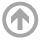Scott Hottovy

# Research Projects

Below is a brief description of my research projects. The projects are presented with the most recent first.
Stochastic Models of the Atmosphere

### A Stochastic Linear Model for the Madden-Julian Oscillation (MJO)

This work is partially supported by Grant NSF DMS-1815061.

The MJO is an intra-seasonal cycle in the tropics, defined as the region around the equator (plus or minus 20 degrees latitude).The MJO is responsible for a significant amount of rainfall over the Indian Ocean and the Maritime Continent. Furthermore, the MJO has an enormous impact on global climate. It impacts tropical cyclones, El Niño-Southern Oscillation, active and break phases of monsoons, midlatitude weather and its predictability, and ocean
biogeochemistry.

This 30 to 90 day cycle is not well understood in the atmospheric science community. Randall 2012, describes the importance for understanding the MJO in the following quote.

"The MJO occurs mainly over the remote tropical oceans and was not discovered until the early 1970s. It strongly influences precipitation over southern Asia and northern Australia, affecting the lives of literally billions of people. It is also believed to influence the timing and intensity of El Ni\~{n}os. Despite its importance, the MJO is perhaps the last type of weather system for which the basic physical mechanisms are not well understood."

My work is focused on using simple stochastic models to accurately capture tropical waves and provide an explanation for the MJO. At their working level these models are linear stochastic differential equations (SDE) with additive noise. Surprisingly, these linear SDEs give a good approximation of the tropical atmosphere. These models will be used to give a theory about how the MJO is is formed (mathematically and physically), and lead to fast testing of different forcing mechanisms (e.g. diurnal cycle, SST fluctuations, ocean coupling).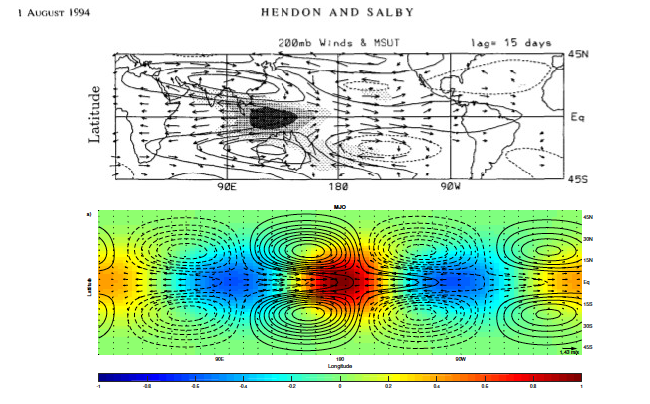Figure: Top, the horizontal structure of the MJO (space time correlations) from Hendon & Salby 1994. Bottom, the horizontal structure of the Stechmann & Hottovy 2017 model's MJO.

#### Selected Paper:

• Stechmann S. N., Hottovy S. 2017: Unified spectrum of tropical rainfall and waves in a simple stochastic modelGeophys. Res. Lett. 44(20). [ pdfsupporting information]

### Single Column Model for Rainfall

A very simple simulation of a rain event is a model of a single cloud in two states: evaporating and precipitating. The cloud accumulates moisture at a rate in the evaporating state until a critical moisture level where the cloud switches dynamics and precipitates. Randomness is introduced into the system to account for fluctuations in the moisture (with white noise) and uncertainty (exponential waiting time once the critical moisture level is reached before switching dynamics). These types of systems exhibit switching dynamics, and are called stochastic hybrid systems, or Markovian switching systems.

### 2D Column Model for the Background Tropical Rainfall

Using the single column model as a starting point, we study systems of interacting clouds both in one-dimension and two. The important questions we study are: Can we use a simple interacting cloud model to capture relevant statistics from nature? What are the important mechanisms in the model? What can we mathematically prove about the models? From here, we can increase complexity of the model and rely more on computational methods.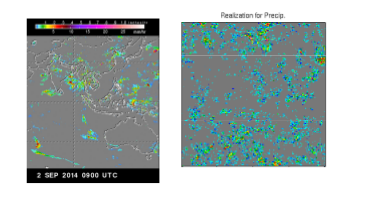Image: On the left is a plot of data from TRMM from NASA. The plot on the right is a realization of the two-dimensional model.

#### Selected Papers:

• Stechmann S N, Hottovy S, 2016: Cloud regimes as phase transitions. Geophys. Res. Lett. 43, 6579-6587. [ pdf ,supporting information , journal ]
• Hottovy, S., Stechmann, S.N. (2015). A spatiotemporal stochastic model for tropical precipitation and water vapor dynamics. Journal of the Atmospheric Sciences, In Press. [pdfjournal]
• Hottovy, S., Stechmann, S.N. (2015). Threshold models for rainfall and convection: Deterministic versus stochastic triggers. SIAM J. of Appl. Math (SIAP), Vol. 75, Iss. 2, pp 861-884, (2015). [pdf,Supp. Mat.journal]

Modeling Brownian Particles

The complexity of systems on a small scale, such as a particle in water, makes modeling every attribute impossible. For example, most processes are driven by noise that has some sort of time correlation, or memory. This memory is usually on a very short time scale. To approximate this, white noise (memoryless) is used. These approximations lead to stochastic differential equations where numerical experiments can be calculated at a much faster rate than the original system.

There are two ways these calculations can be viewed and carried out. One is using the stochastic differential equation directly and running a slight modification of an ODE numerical solver. The text,Numerical Solution of Stochastic Differential Equations by P.E. Kloeden and E. Platen, is a good resource for these trajectory driven numerics. A problem of study is the rate at which a particle, trapped by a biharmonic potential, jumps between the two wells (see right).

Another advantage of modeling with SDEs is the Fokker-Planck (or Forward Kolmogorov) and Backward Kolmogorov partial differential equations associated with a given SDE (under some assumptions). These two PDEs give an equation for the probability density function of the process. This opens up a whole branch of mathematics that is well studied. Along with the theoretical tools, one can also numerically solve these PDEs.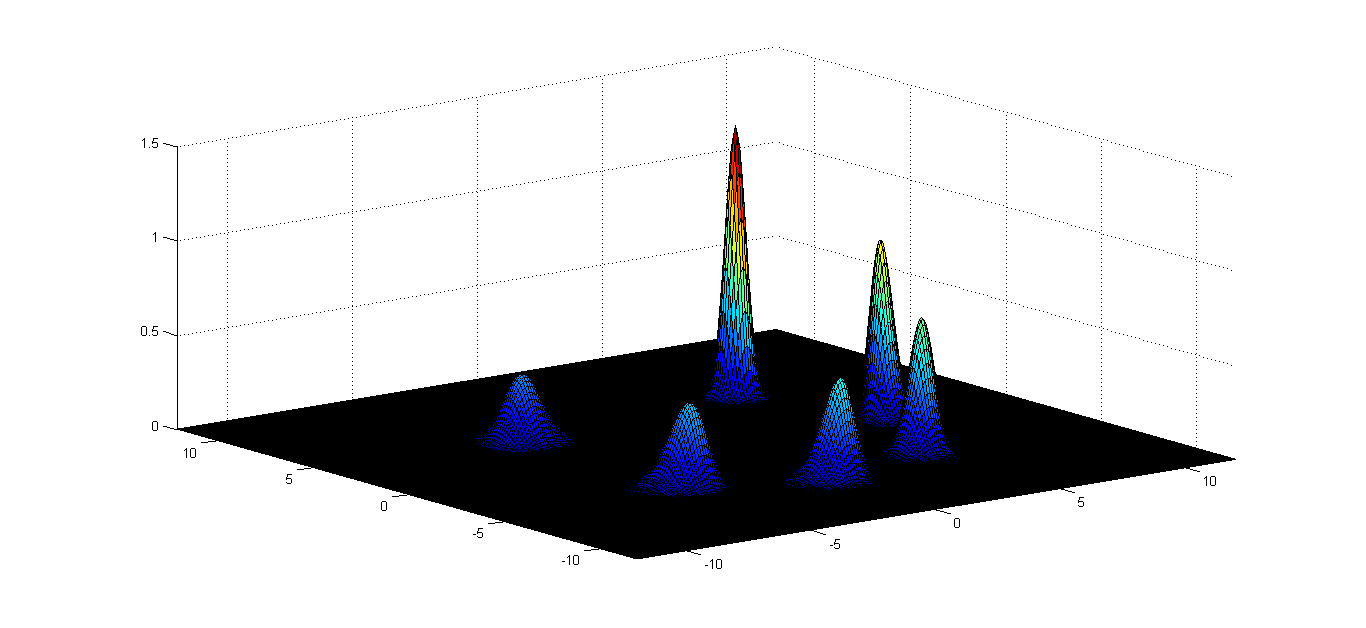Image: Evolution of the probability density function using the Fokker-Planck Equation simulated in MATLAB.

Selected Papers:

• Pesce, G., McDaniel, A., Hottovy, S., Wehr, J. & Volpe, G. (2013). Stratonovich-to-Itô transition in noisy systems with multiplicative feedback. Nature Communications, 4, 2733. [ArXivjournal]
• The Fokker-Planck Equationby Scott Hottovy for Numerical PDE course at the University of Arizona
• This is a case study of a numerical PDE paper, B. F. Spencer Jr. and L. A. Bergman. On the numerical solution of the Fokker-Planck equation for nonlinear stochastic system. Nonlinear Dynamics, 4:357-372, 1993.
•
Small mass approximation

This research considers stochastic differential equations (SDE) arising in physical systems. An example of such a system is a small but dense molecule, on the order of 1 micrometer, in water. To make things easier we only write down one equation, using Newton's second law, for the molecule in question and include a random forcing term (with some assumptions) to account for the collisions. The result is a second order stochastic differential equation called the Langevin equation. For a more descriptive derivation, see Toda, Kubo, and Saitô's Statistical Mechanics book.

Within this class of equations I am interested in the relation between the small mass approximation and the different forces (random and non) influencing the particle. My work includes analysis techniques from the theory of stochastic processes, multiscale analysis, and probability as well as numerical analysis to simulate trajectories of the particle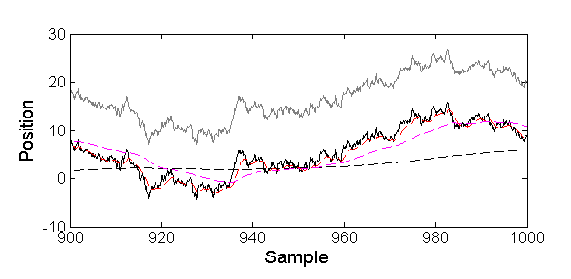Image: The dotted lines are trajectories of the Langevin SDE, converging to the Smoluchowski-Kramers approximation (solid black line). The grey line is an incorrect approximation.

#### Selected Papers:

• Hottovy, S., McDaniel, A., Volpe, G., & Wehr, J. (2014). The Smoluchowski-Kramers limit of stochastic differential equations with arbitrary state-dependent friction. Communications in Mathematical Physics, 336(3), 1259-1283. [ArXivjournal
• Hottovy, S., Volpe, G. & Wehr, J. (2012). Thermophoresis^M of Brownian particles driven by coloured noise. EPL, 99(6), 60002. [ArXivjournal]